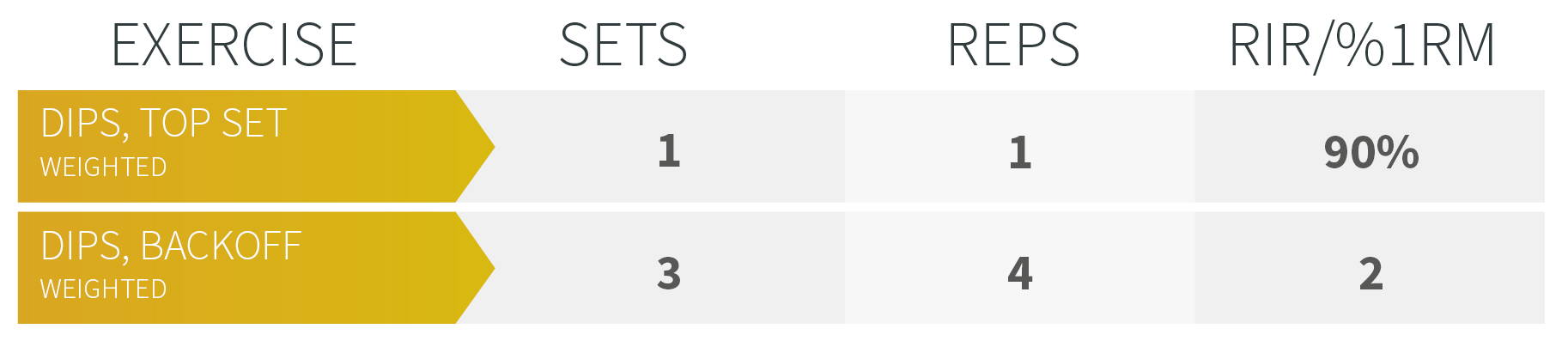# HOW TO CALCULATE YOUR 1RM

If you do not know your 1RM, you can calculate an approximation of your e1RM (estimated) with the help of different formulas. Below are 3 different formulas that give you 3 different but similar results. The closer your set with which you calculate the e1RM is to your real 1RM, meaning the fewer repetitions the set has, the more accurate the results are. So if possible, calculate with a set of fewer than 5 repetitions.

EPLY:1RM = WEIGHT + (WEIGHT × REPETITIONS ÷ 30)

BRZYCKI:1RM = WEIGHT × 36 ÷ (37 − REPETITIONS)

LANDER: 1RM = 100 × WEIGHT ÷ (101,3 − 2,67123 × REPETITIONS)

The higher your 1RM is, so the more advanced you are, the more inaccurate these values become. Take these values as an orientation. Besides, you must, of course, include your pre-fatigue and daily condition in the weight selection. The working weight may then vary by 10kg or more for the same set on two different days. This is normal since we are humans and no machines.

In the King Of Weighted Program, we work with two different ways to display intensities. Once with absolute intensities in % of the 1RM or e1RM for the top singles and with relative intensities in RIR that take into account the daily form. The top sets are given in absolute % so that we can visualize progress and plan better. Also, the mental attitude to the set is usually better with non-variable intensity in the top sets, which can affect the performance. The backoff sets are then specified in RIR.EXAMPLE FOR THE GIVEN PLAN

e1RM CALCULATION MICHA SCHULZ

STRONGEST SET DIPS: 5x100KG

EPLY: 1RM = 100 + (100 × 5 ÷ 30) = 116,7kg

BRZYCKI: 1RM = 100 × 36 ÷ (37 − 5) = 112,5kg

LANDER: 1RM = 100 × 100 ÷ (101,3 − 2,67123 × 5) = 113,7kg

If I put in my strongest set in the formula, I receive an e1RM of 113-117kg. My strongest dip ever executed in a competition was 120kg. This means the EPLEY formular was pretty accurate. So if you don't know your real max, you can easily calculate with the e1RM from the formulas:

113,7kg | 116,7kg × 0,9 = 102kg | 105kg

For the backoff sets the intensity is give in RIR. So if we have no experience with RIR, we can use the table and get a value of 83,7% to calculate our working weight:

113,7 | 116,7 × 0,837 = 95kg | 98kg